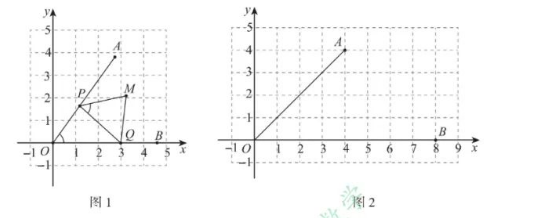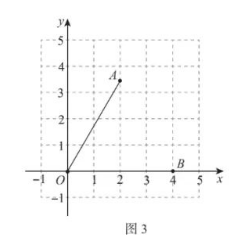(1) 如图 2, 已知点 $A(4,4), B(8,0)$, 点 $P$ 与点 $A$ 重合.
① 当点 $Q$ 是线段 $O B$ 中点时, 在 $M_1(4,2), M_2(6,2)$ 中, 其中是线段 $P Q$ 的 “关联点” 的是
② 已知点 $M(8,4)$ 是线段 $P Q$ 的 “关联点”, 则点 $Q$ 的坐标是(2) 如图 3, 已知 $O A=O B=4, \angle A O B=60^{\circ}$.
①当点 $P$ 与点 $A$ 重合, 点 $Q$ 在线段 $O B$ 上运动时 (点 $Q$ 不与点 $O$ 重合), 若点 $M$ 是线 段 $P Q$ 的 “关联点”, 求证: $B M / / O A$;
②当点 $P, Q$ 分別在线段 $O A, O B$ 上运动时, 直接写出线段 $P Q$ 的 “关联点” $M$ 形成的 区域的周长.①点击 首页查看更多试卷和试题 , 点击查看 本题所在试卷